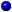SYLLABUS  Previous: 9.1 Typesetting with TEX  Up: 9 PROBLEM BASED LEARNING  Next: 9.3 Parameters and switches

## 9.2 Programming in JAVA

Slide : [ Java || JBONE tree - names || JAVA tutorial ]

Numerical schemes submitted from the Java window are automatically inserted in the JBONE source code (e.g. solution 2.01) and have first to be compiled on our server before you can download and execute them locally in your browser. This page introduces a limited number of Java commands you may need to carry out your assignments. More details concerning the JBONE applet can be found in the program tree, the name index and finally in the program listing. For a complete tutorial in Java programming, consult the excellent course from Sun Microsystems.JBONE = Java Bed for ONE dimensional problems.

The JBONE applet is a wrapper to perform a stepwise evolution of functions, i.e. solving time-dependent differential equations using algorithms that can schematically be written as
1. At `time=0` use the initial condition to initialize the functions `f0[i], f[i],g[i]`
2. Plot `f` (black curve), `f0` (grey), and `g` (blue).
3. Use the Java-code submitted in an assignment to calculate the new values for `fp` after a small time step in terms of present `f` and sometimes past values `fm`.
4. Shift the time levels `time=time+timeStep` and the solution arrays `fm=f; f=fp`.
5. Goto 2 until finished.
Only the third step can be modified by the user in the Java window; for example, a simple loop
```   double scale = runData.getParamValue("UserDouble");
for (int i=0; i<=n; i++) {
fp[i]=scale*f[i];
}
```
executes an artificial evolution, where the next value `fp` is obtained from a simple scaling of the present value `f`. Remember that you have to force your browser to reload the applet after each modification, or prevent your browser from using the older version that is often stored in the browser data cache.JBONE variables.

From the list of run parameters (a Java object called runData), the JBONE applet first defines local variables (double = 16 digits precision real, int = up to 9 digits signed integer) using statements
 advection vel `double vel =runData.getParamValue("Velocity");` diffusion coeff `double diff =runData.getParamValue("Diffusion");` dispersion coeff `double disp =runData.getParamValue("Dispersion");` time step `double step =runData.getParamValue("TimeStep")();` number mesh pts `int n =runData.getParamValueInt("MeshPoints");` number particles `int N =runData.getParamValueInt("Walkers");` implicit time `double theta=runData.getParamValue("TimeTheta");` tunable quadrat. `double tune =runData.getParamValue("TuneQuad");` IC amplitude `double ampl =runData.getParamValueInt("ICAmplitude");` IC position `double pos =runData.getParamValueInt("ICPosition");` IC width `double width=runData.getParamValueInt("ICWidth");` IC wavelength `double wavel=runData.getParamValueInt("ICWavelength");` total run time `double rTime=runData.getParamValue("RunTime");` lower value axis `double x0 =runData.getParamValue("MeshLeft");` length of axis `double len =runData.getParamValue("MeshLength");` user defined int `int myInt=runData.getParamValueInt("PhysDataCase");` user defined dbl `double myDbl=runData.getParamValue("PhysDataValue");`

and computes the evolution of the variables and arrays defining the solution (an object called solution)
 time in the simulation `double time;` last index of solution `int n = x.length-1;` mesh points, intervals `double[] x,dx;` solution functions `double[] f0,f,g;` `Complex[] h, s;` old, present, future `double[] fm,f,fp;` derivatives `double[] dfm,df,dfp;` current positions `double[] particlePosition;`

Note that in Java (as in C and C++), the index of arrays starts with zero (`x`) and finishes with an index lower by one element less than its size (`x[x.length-1]`).Debugging.

Having corrected all the compiler errors does unfortunately not mean that your scheme immediately behaves the way you want... You may have to monitor the value of the quantities you defined, using statements of the form
```/* the mistake dividing by zero has been commented out for debugging
double error = fp[n]/0;
*/
System.out.println("Value fp["+i+"] = "+fp[i]);
```
This example will print the values of the array `fp` to the Java Console of your browser (with Netscape select Communicator + Tools + Java Console, with Explorer first select Tool + Internet Options + Advanced + Java console enabled and then View + Java Console). From the values that are printed after a single step, it is possible to track down most of the mistakes. Another debuging strategy is to temporarily des-activate a portion of your program, using the `\* Java comment delimiters *\` that can extend over several lines.Common errors.

Avoid the most common difficulties when you start programming for your assignments
• Every new variable that is not explicitly listed in the variable index) has to be declared; in particular, memory must be allocated for arrays and objects using the command `new`
```  int i = 3;                // Declare i as an integer
double[] c;               // Declare c[] array 16 digits nbrs
c = new double[i];        // Memory for c, c, c
BandMatrix  A;            // Declare A as a BandMatrix object
A = new BandMatrix(3,10); // Memory for 3 bands with 10 doubles
```
• Accessing the element `c` before the memory has been attributed with a `new` statement leads to the infamous `java.lang.NullPointerException` error; using `c` throws an `java.lang.ArrayIndexOutOfBoundsException:3`, since the array is accessed outside its valid range 0,1,2.

• In Java, the assigning equal sign is denoted by as single `=` whereas the comparing equal sign by a double `==`
```  int a = 42;
if (a == 17) System.out.println("a is equal to 17");
if (a != 17) System.out.println("a is not equal to 17");
```
will print the text "`a is not equal to 17`" to the Java Console.

SYLLABUS  Previous: 9.1 Typesetting with TEX  Up: 9 PROBLEM BASED LEARNING  Next: 9.3 Parameters and switches